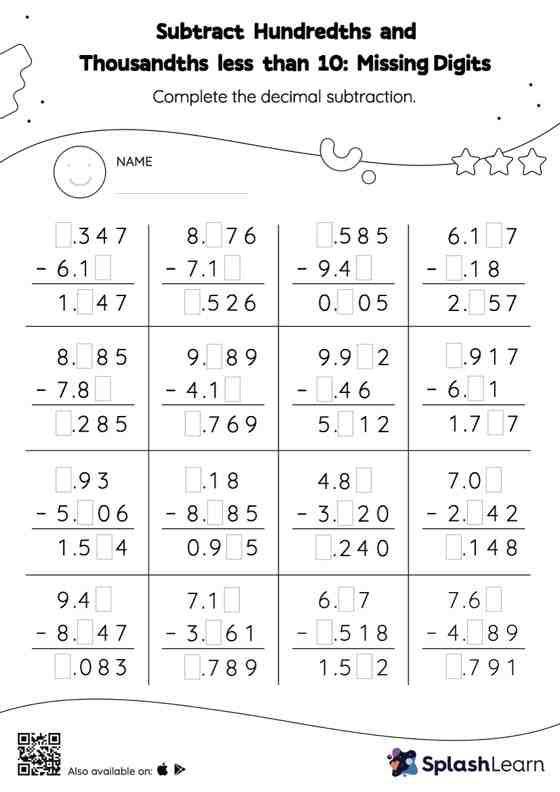# Subtract Hundredths and Thousandths less than 10: Missing Digits Worksheet

Home > Subtract Hundredths and Thousandths less than 10: Missing DigitsGive your little one the opportunity to dive deeper into subtraction with this subtract hundredths and thousandths less than 10 worksheet. When subtracting decimals on this worksheet, students align the decimal points and use zero as a placeholder. Then to find the missing number in the subtract hundredths and thousandths less than 10 worksheet, students then apply the relationship between addition and subtraction.